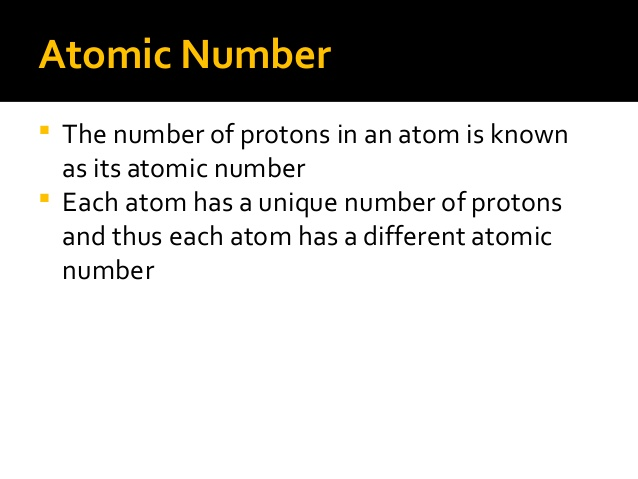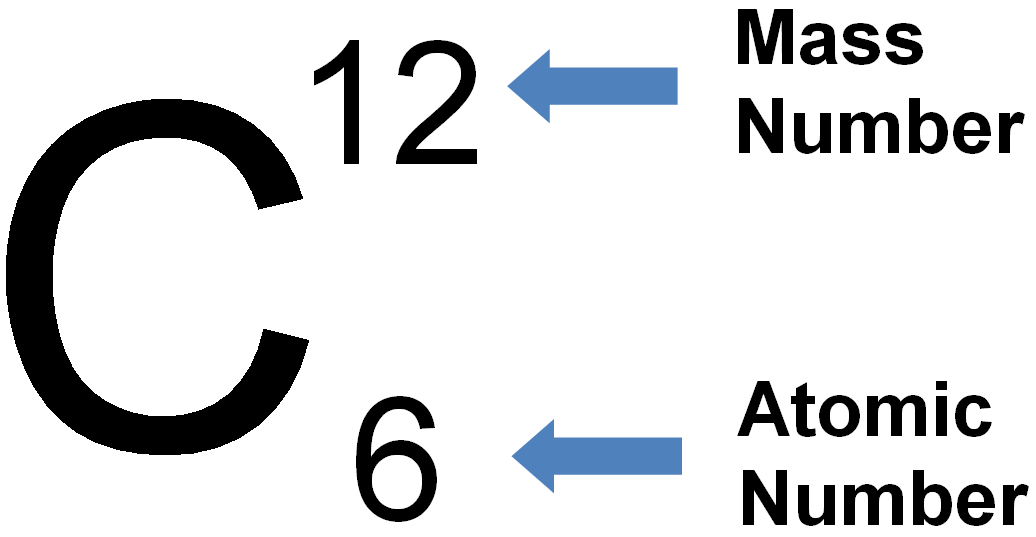# What Is Atomic Number And Mass Number?

Hello Friends!

In this article, you will get the detailed knowledge of Atomic Number & Mass Number. The atomic number is the total numbers of the of protons in the nucleus present of an atom which gives you atom number and is represented by capital Z.

The combination of neutrons and protons that gives you a mass number of an atom, a combination of both are called nucleons.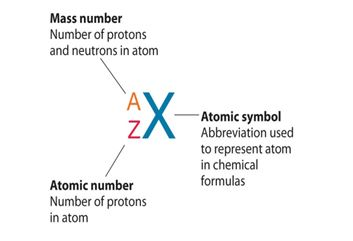## Atomic Number Periodic Table

We are providing you here atomic number periodic table.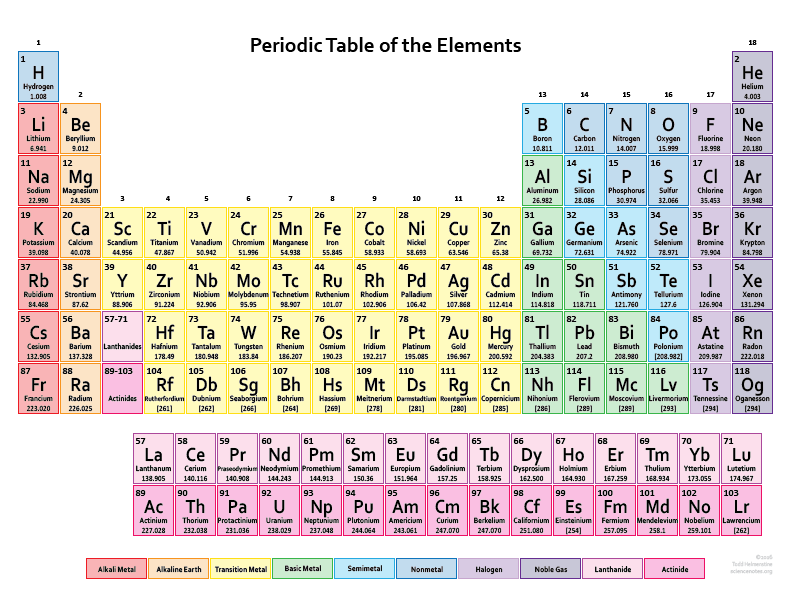## How To Find Atomic Number

For any element:

Number of Protons = Atomic Number

Number of Electrons = Number of Protons = Atomic Number

Number of Neutrons = Mass Number – Atomic Number

For krypton:

Number of Protons = Atomic Number = 36

Number of Electrons = Number of Protons = Atomic Number = 36

Number of Neutrons = Mass Number – Atomic Number = 84 – 36 = 48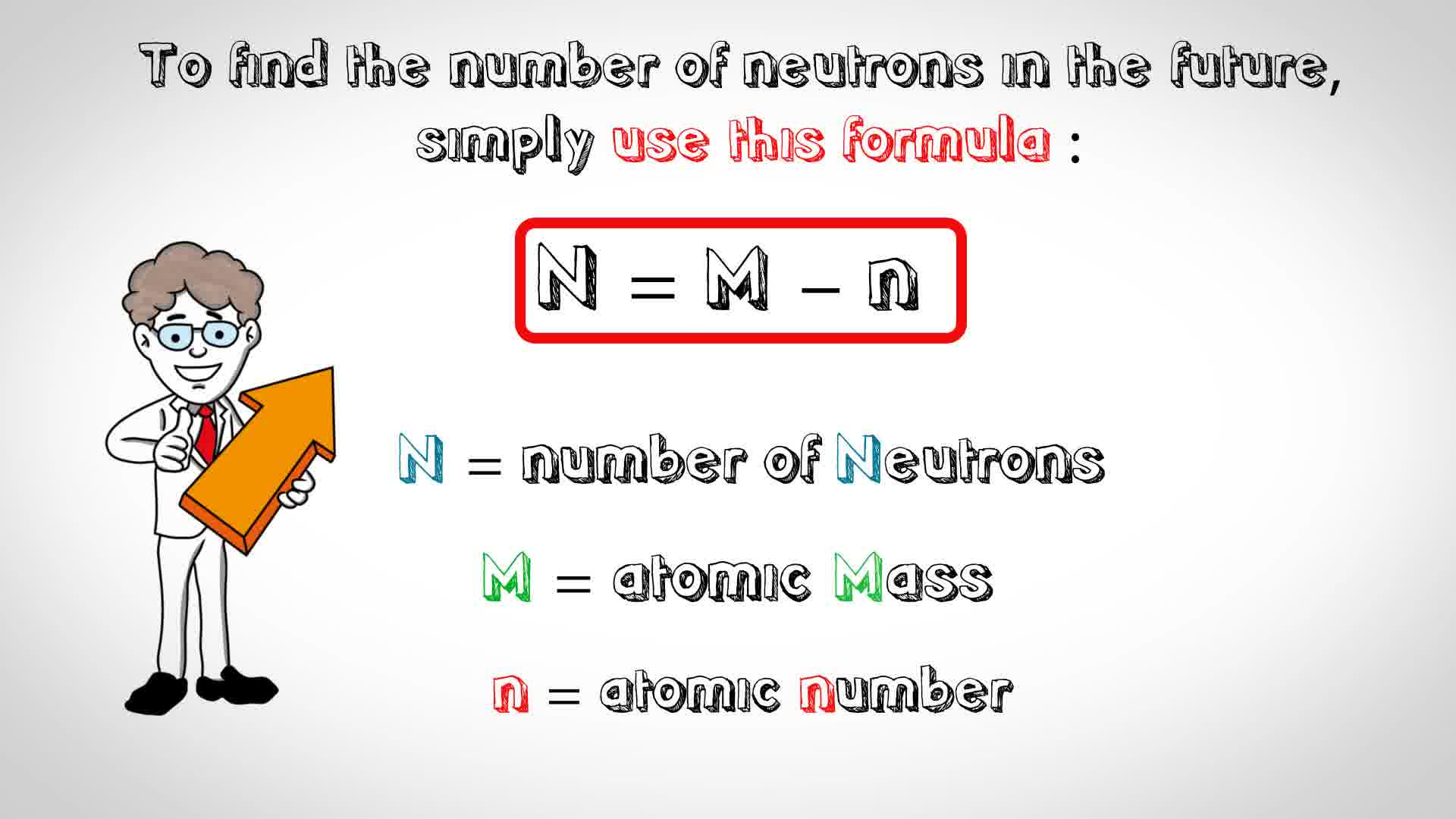## Atomic Number Examples

Example for the atomic number will be cleared to you in the image given below.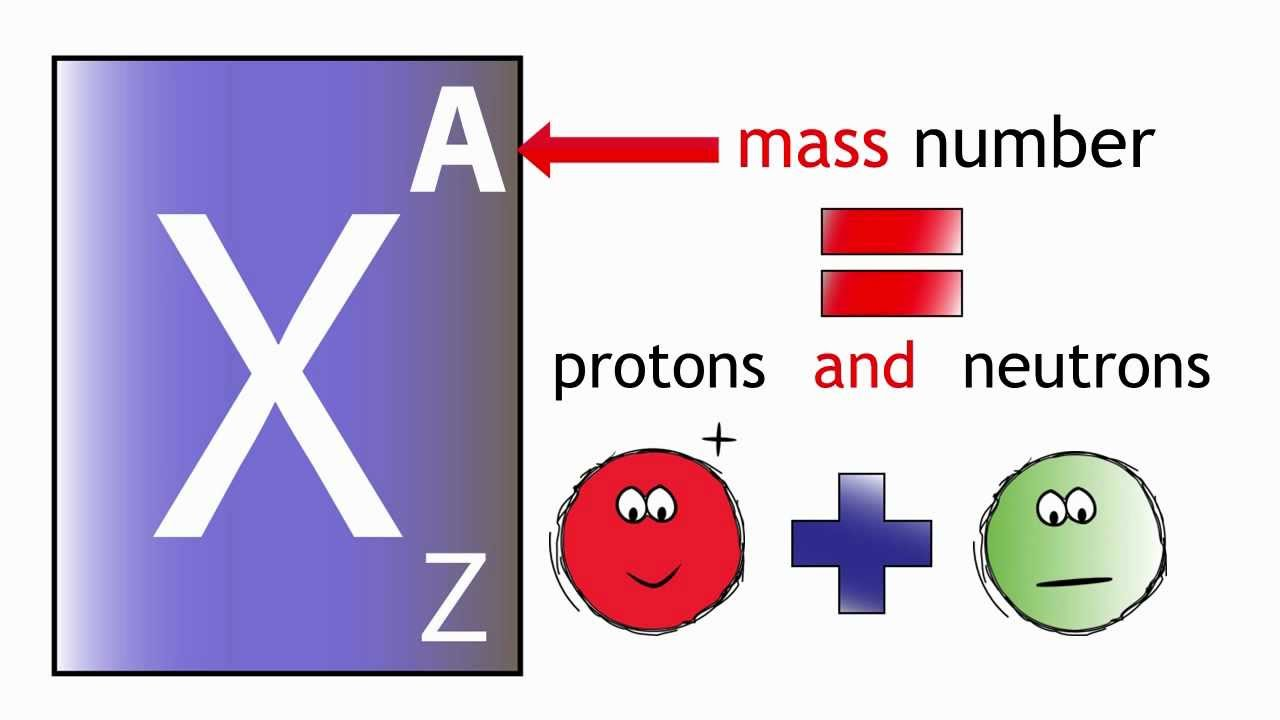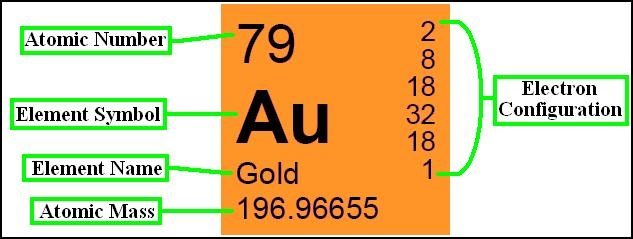## What Is The Atomic Number Of An Element?

The atomic number is the total numbers of the of protons in the nucleus present of an atom which gives you atom number.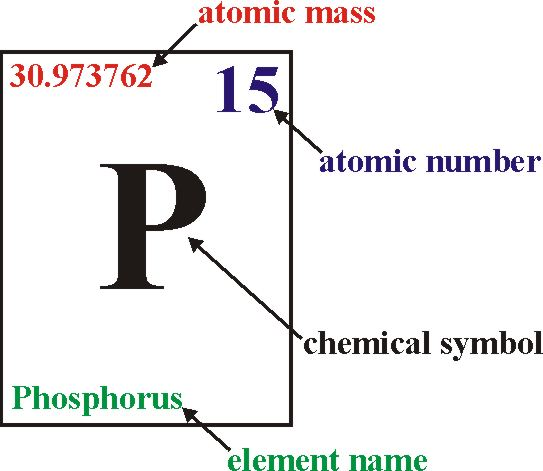## What Is The Definition Of Atomic Number?

To know this above mention question than have a look on image given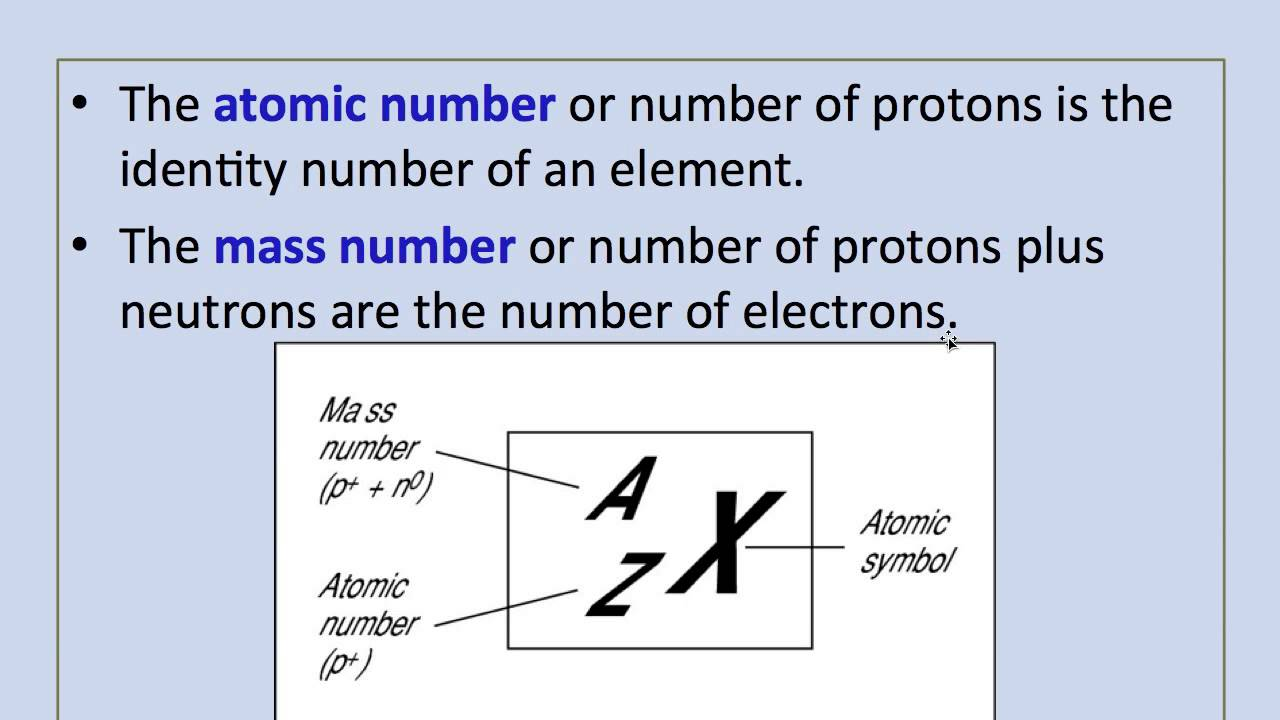## What Is An Atomic Number In Chemistry?